# mgcViz basics

The mgcViz R package (Fasiolo et al, 2018) offers visual tools for Generalized Additive Models (GAMs). The visualizations provided by mgcViz differs from those implemented in mgcv, in that most of the plots are based on ggplot2's powerful layering system. This has been implemented by wrapping several ggplot2 layers and integrating them with computations specific to GAM models. Further, mgcViz uses binning and/or sub-sampling to produce plots that can scale to large datasets ($$n \approx 10^7$$), and offers a variety of new methods for visual model checking/selection.

This document introduces the following categories of visualizations:

1. smooth and parametric effect plots: layered plots based on ggplot2 and interactive 3d visualizations based on the rgl library;

2. model checks: interactive QQ-plots, traditional residuals plots and layered residuals checks along one or two covariates;

3. special plots: differences-between-smooths plots in 1 or 2D and plotting slices of multidimensional smooth effects.

## Layered smooth effect plots

Here we describe effect-specific plotting methods and then we move to the plot.gamViz function, which wraps several effect plots together.

### Effect-specific plots

library(mgcViz)
n  <- 1e3
dat <- data.frame("x1" = rnorm(n), "x2" = rnorm(n), "x3" = rnorm(n))
dat$y <- with(dat, sin(x1) + 0.5*x2^2 + 0.2*x3 + pmax(x2, 0.2) * rnorm(n)) b <- gam(y ~ s(x1) + s(x2) + x3, data = dat, method = "REML")  Now we convert the fitted object to the gamViz class. Doing this allows us to use the tools offered by mgcViz and to save quite a lot of time when producing multiple plots using the same fitted GAM model. b <- getViz(b)  We extract the first smooth component using the sm function and we plot it. The resulting o object contains, among other things, a ggplot object. This allows us to add several visual layers. o <- plot( sm(b, 1) ) o + l_fitLine(colour = "red") + l_rug(mapping = aes(x=x, y=y), alpha = 0.8) + l_ciLine(mul = 5, colour = "blue", linetype = 2) + l_points(shape = 19, size = 1, alpha = 0.1) + theme_classic()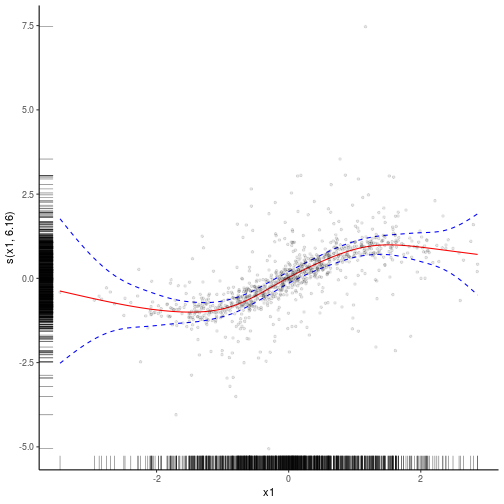We added the fitted smooth effect, rugs on the x and y axes, confidence lines at 5 standard deviations, partial residual points and we changed the plotting theme to ggplot2::theme_classic. Functions such as l_fitLine or l_rug are effect-specific layers. To see all the layers available for each effect plot we do: listLayers(o)  ##  "l_ciLine" "l_ciPoly" "l_dens2D" "l_fitDens" "l_fitLine" "l_points" ##  "l_rug" "l_simLine"  Similar methods exist for 2D smooth effect plots, for instance if we fit: b <- gam(y ~ s(x1, x2) + x3, data = dat, method = "REML") b <- getViz(b)  we can do plot(sm(b, 1)) + l_fitRaster() + l_fitContour() + l_points()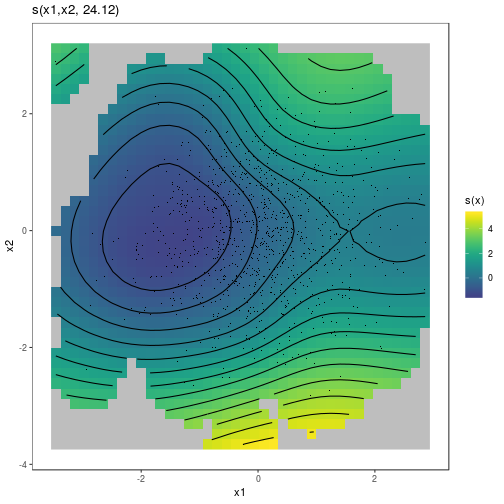We can extract the parametric effect x3 using the pterm function (which is the parametric equivalent of sm). We can then plot the two effects on a grid using the gridPrint function: gridPrint(plot(sm(b, 1)) + l_fitRaster() + l_fitContour() + labs(title = NULL) + guides(fill=FALSE), plot(pterm(b, 1)) + l_ciPoly() + l_fitLine(), ncol = 2)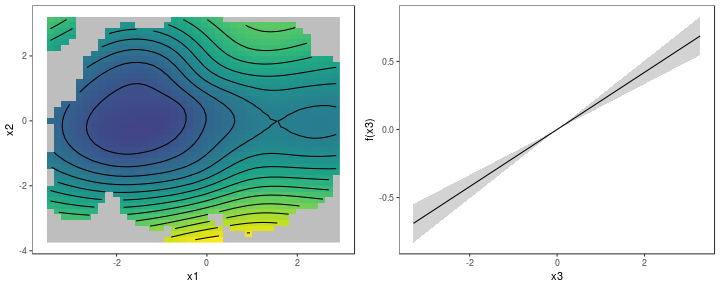If needed, we can convert a gamViz object back to its original form by doing: b <- getGam(b) class(b)  ##  "gam" "glm" "lm"  The only reason to do so might be to save some memory (gamViz objects store some extra objects). ### The plot.gamViz method The plot.gamViz is the mgcViz's equivalent of mgcv::plot.gam. This function wraps together the plotting methods related to each specific smooth or parametric effect, which can save time when doing GAM modelling. Consider this model: dat <- gamSim(1,n=1e3,dist="normal",scale=2) dat$fac <- as.factor( sample(letters[1:6], nrow(dat), replace = TRUE) )
b <- gam(y~s(x0)+s(x1, x2)+s(x3)+fac, data=dat)


To plot all the effects we do:

b <- getViz(b)
print(plot(b, allTerms = T), pages = 1) # Calls print.plotGam()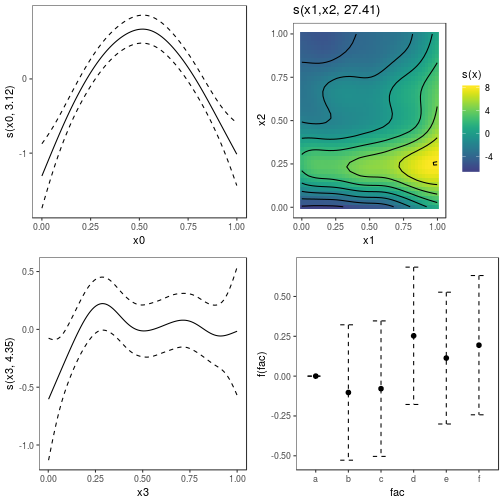Here plot calls plot.gamViz, and setting allTerms = TRUE makes so that also the parametric terms are plotted. We are calling print (which uses the print.plotGam method) explicitly, because we want to put all plots on one page. Alternatively we could have simply done:

plot(b)


which plots only the smooth effects, diplaying one on each page.

Notice that plot.gamViz returns an object of class plotGam, which is initially empty. The layers in the previous plots (e.g. the rug and the confidence interval lines) have been added by print.plotGam, which adds some default layers to empty plotGam objects. This can be avoided by setting addLay = FALSE in the call to print.plotGam. A plotGam object in considered not empty if we added objects of class gamLayer to it, for instance:

pl <- plot(b, allTerms = T) + l_points() + l_fitLine(linetype = 3) + l_fitContour() +
l_ciLine(colour = 2) + l_ciBar() + l_fitPoints(size = 1, col = 2) + theme_get() + labs(title = NULL)
pl\$empty # FALSE: because we added gamLayers

##  FALSE

print(pl, pages = 1)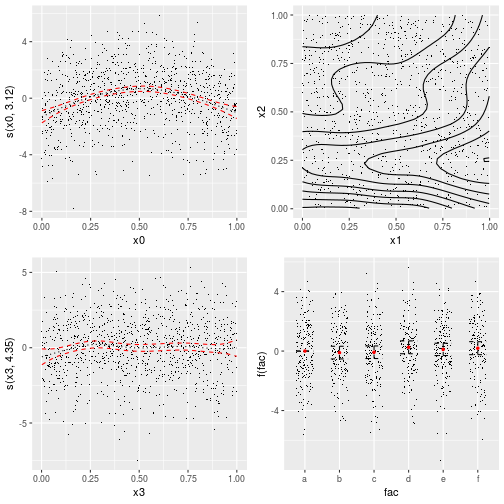Here all the functions starting with l_ return gamLayer objects. Notice that some layers are not relevant to all smooths. For instance, l_fitContour is added only to the second smooth. The +.plotGam method automatically adds each layer only to compatible effect plots.

We can plot individuals effects by using the select arguments. For instance:

plot(b, select = 1)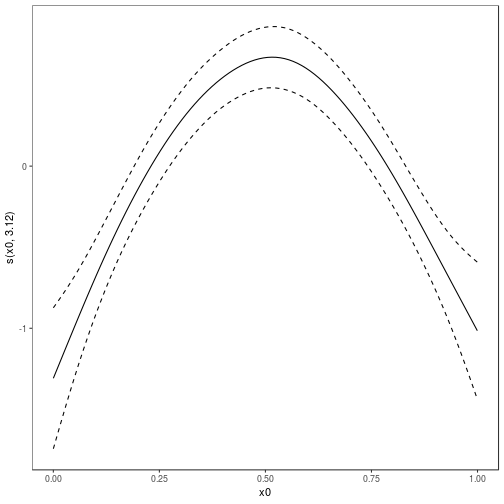where only the default layers are added. Obviously we can have our custom layers instead:

plot(b, select = 1) + l_dens(type = "cond") + l_fitLine() + l_ciLine()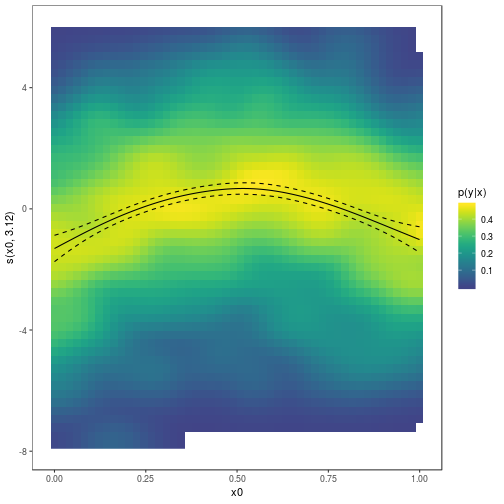where the l_dens layer represents the conditional density of the partial residuals. Parametric effects always come after smooth or random effects, hence to plot the factor effect we do:

plot(b, allTerms = TRUE, select = 4) + geom_hline(yintercept = 0)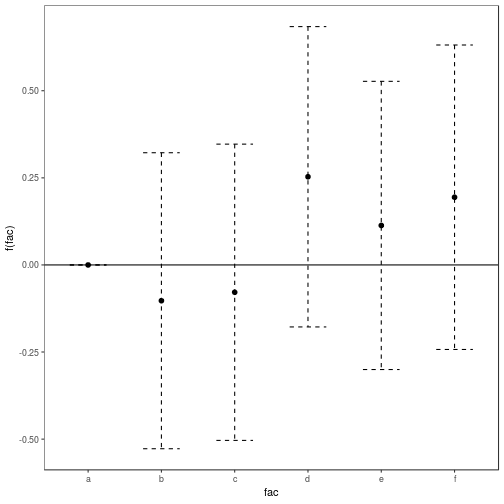### Interactive rgl smooth effect plots

mgcViz provides tools for generating interactive plots of multidimensional smooths via the rgl R package. Here is an example where we are plotting a 2D slice of a 3D smooth effect, with confidence surfaces:

library(mgcViz)
n <- 500
x <- rnorm(n); y <- rnorm(n); z <- rnorm(n)
ob <- (x-z)^2 + (y-z)^2 + rnorm(n)
b <- gam(ob ~ s(x, y, z))
b <- getViz(b)

plotRGL(sm(b, 1), fix = c("z" = 0), residuals = TRUE)